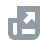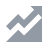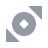Markets
Futures
Earn
More
Cancel
FAQAccount FunctionsTutorialBinance Fan TokenBinance EarnCrypto Deposit/WithdrawalCrypto DerivativesFinanceAPISecurityBinance ConvertNFTVIP
Home
Support Center
FAQ
Crypto Derivatives
Futures Contracts
Introduction to Binance Futures
Introduction to Binance Futures Funding Rates

# Introduction to Binance Futures Funding Rates

2020-06-09 11:21

## 1. What is the Funding Rate?

Funding rates are periodical cash flows exchanged between long- and short-position holders. The absolute amount per lot will be based on the difference between the perpetual Futures and index prices. When the market is bullish, the funding rate is usually positive. Traders with long positions will pay traders on the short side. Conversely, the funding rate will usually be negative when the market is bearish, and traders with short positions will pay traders on the long side.
Please note that Binance only helps facilitate the exchange of funding amounts between long- and short-position holders. Binance does not charge any service fees.

## 2. Why is the Funding Rate important?

Unlike traditional Futures, perpetual Futures contracts have no expiration date. Traders can hold their positions perpetually unless they are liquidated. To ensure perpetual contracts’ prices correspond to their underlying markets, crypto exchanges created a mechanism known as Funding Rate.
The funding rate primarily helps perpetual contracts’ prices converge to the underlying asset's price. With sufficient liquidity, trading perpetual contracts are very similar to trading in the spot market.
As such, crypto exchanges created a mechanism to ensure that perpetual contract prices correspond to the index. This is known as the Funding Rate.

## 3. How are Funding Rates calculated on Binance?

Funding Amounts are calculated using the following formula:
Funding Amount = Nominal Value of Positions * Funding Rate
Where
• Nominal Value of Positions = Mark Price * Size of a Contract (for USDⓈ-Margined contracts)
• Contract Multiplier * Size of a Contract/Mark Price (for Coin-Margined contracts)
Funding payments generally occur every 8 hours at 00:00 UTC, 08:00 UTC, and 16:00 UTC for all Binance Futures perpetual contracts. Traders are only liable for funding payments in either direction if they have open positions at the pre-specified funding times. You are not liable for any funding if you do not have a position. If you close your position before the funding time, you will not pay or receive any funding.
There is a 1-minute deviation in the actual funding fee transaction time. For example, when a trader opens a position at 08:00:59 UTC, the funding fee could still apply to the trader (either paying or receiving the funding fee).
You can view the Funding Rates and the countdown timer to the next funding on the Binance Futures interface above the candlestick chart:
Please note that the funding rate shown represents an estimation of the last 8 hours of the premium index. For example, from 09:00 UTC, the funding rate calculation uses the premium index dataset from 01:00 UTC to 09:00 UTC (rather than from 08:00 UTC to 09:00 UTC).

## 4. What determines the Funding Rate?

There are two components to the Funding Rate: the Interest Rate and the Premium. Binance uses a flat interest rate, with the assumption that holding cash equivalent returns a higher interest than BTC equivalent.
On Binance Futures, the interest rate is fixed at 0.03% daily by default (0.01% per funding interval since funding occurs every 8 hours). Please note that this doesn’t apply to certain contracts, such as BNBUSDT, BNBBUSD and ETHBTC’s interest rates are at 0%.
There may be a significant difference in price between the perpetual contract and the Mark Price. On such occasions, a Premium Index will be used to enforce price convergence between the two markets. The Premium Index history can be viewed here. It is calculated separately for every contract:
Premium Index (P) = [Max(0, Impact Bid Price - Price Index ) - Max(0, Price Index - Impact Ask Price)] / Price Index
Impact Bid Price = The average fill price to execute the Impact Margin Notional on the Bid Price
Impact Ask Price = The average fill price to execute the Impact Margin Notional on the Ask Price
• Price Index is the weighted average value of the underlying asset listed on major spot exchanges.
• The Impact Margin Notional (IMN) is used to locate the average Impact Bid or Ask price in the order book. IMN for USDⓈ-Margined contracts is the notional available to trade with 200 USDT worth of margin (price quote in USDT). IMN for COIN-Margined contracts is the notional available to trade with 200 USD worth of margin (price quote in USD).
Impact Margin Notional (IMN) = 200 USDT / Initial margin rate at the maximum leverage level
For example, if the maximum leverage of BNBUSDT perpetual contract is 20x, and its corresponding Initial Margin Rate is 5%, then the Impact Margin Notional (IMN) is 4,000 USDT (200 USDT / 5%), and the system will take an IMN of 4,000 USDT every minute in the order book to measure the average Impact Bid/Ask price.

## 5. How to access the real-time and historical Funding Rates?

You can view the real-time and historical Funding Rates by clicking [Information] - [Funding Rate History]. Alternatively, click here directly.

2. Click the manage button to go to [Preference].
3. Go to the [Notification] tab to toggle on the [Funding Fee Trigger] button. You can also customize the Funding Rate charges percentage between 0.0001%~0.75%. Currently, it is defaulted to 0.25%, meaning you will be notified when the expected Funding Rate charges reach 0.25%.
Important note: You will be notified via email / in-app notification. This function serves as a risk warning and Binance cannot guarantee timely delivery. You agree that during your use of the Service, under certain circumstances (including but not limited to personal network congestion and poor network environment), you may not be able to receive or receive delayed reminders. Binance reserves the right and has no obligation to deliver notifications.

## 7. How to calculate the Funding Rate?

### Step 1. Find the Impact Bid/Ask Price Series in a given funding period

Let’s use the following Bid-side order book for a USDⓈ-Margined contract as an example:
If multiplier *∑px*qx > IMN in Level x and multiplier * ∑px-1*qx-1 < IMN in Level x-1, then we can find the Impact Bid Price from the Level x order book:
Impact bid price =IMN / [(IMN-multiplier *∑px-1*qx-1)/px+multiplier * ∑qx-1]
*IMN: Impact Margin Notional
To get the Impact Bid/Ask Price Series, the system performs the above methodology over the order book snapshots in this funding period:
The Ask order book is summarized as below:
*Assume the maximum leverage of BNBUSDT perpetual contract is 125x and the contract default Impact Margin Notional is 25,000 USDT.
From the table above, we get the following figures:
• Price at Level x = 279.71
• Accumulated quote notional quantity at Level x - 1 = 22,704.65
• Accumulated base quantity at Level x-1 = 41.86 + 6.26 + 1.42 + 31.64 = 81.18
Substituting into the formula:
Impact Ask Price = IMN / [(IMN-multiplier * ∑px-1 * qx-1)/px+multiplier * ∑qx-1]
= 25,000 / [(25,000 - 22,704.65) / 279.71 + 81.18]
= 279.69 USDT
Analysis:
• The corresponding quantity when it reaches IMN at Level x: (IMN-multiplier *∑px-1*qx-1) / px = (25,000 - 22,704.65 ) / 279.71 = 8.206
• Accumulated base quantity when it reaches IMN: 8.206 + 81.18 = 89.386
• Impact Ask Price = 25,000 / 89.386 = 279.69

### Step 2. Find the Premium Index Series in the observed funding period

Binance calculates the Premium Index every 5 seconds (12 premium index data points in a minute).
The number of data points used to calculate the Funding Rate n = 60/5 * 60 * hours of the funding interval
For example, the Funding Rate is calculated by taking the time-weighted average across all 5,760 premium index data points for the 8-hour funding interval.
Click to view the Premium Index History.
Premium Index (P) = [ Max(0, Impact Bid Price - Price Index ) - Max(0, Price Index - Impact Ask Price)] / Price Index
(Max(0,bpn-ipn)-Max(0,ipn-apn))/ipn

### Step 3. Time-to-funding weighted Average of Premium Index of the observed funding period

Use the Premium Index Series in this funding period (from step 2), we substitute it to the Average Premium Index formula:
n = 60/5 * 60 * 8 = 5,760 (Assume the funding interval is 8 hours)

### Step 4. Calculate the Funding Rate

The Funding Rate is then calculated with this 8-Hour Interest Rate Component and the 8-Hour Premium Component. A +/- 0.05% damper is also added. For example, the Funding Rates calculated from 00:00 - 08:00 are exchanged at 08:00.
The Funding Rate formula:
Funding Rate (F) = Average Premium Index (P) + clamp (interest rate - Premium Index (P), 0.05%, -0.05%)
*Premium Index (P) here refers to the current average
Note:
The function clamp (x, min, max) means that if (x < min), then x = min; if (x > max), then x = max; if max ≥ x ≥ min, then return x.
In other words, as long as the Premium Index is between -0.04% to 0.06%, the Funding Rate will equal 0.01% (the Interest Rate).
If (Interest Rate (I) - Premium Index (P)) is within +/-0.05%, then F = P + (I - P) = I. In other words, the Funding Rate will equal the Interest Rate.
Example 1:
Time stamp: 2020-08-27 20:00:00 UTC
Price Index: 11,312.66 USDT
Impact Bid Price: 11,316.83 USDT
Premium Index(P) = Max(0, Impact Bid Price − Price Index ) − Max(0, Price Index − Impact Ask Price) / Price Index
=Max(0, 11,316.83 - 11,312.66) - Max(0,11,312.66 - 11317.66) / 11,312.66
= (4.17 - 0) / 11,312.66
= 0.0369%
*Please note that this example is within the funding period 16:00 - 24:00 (UTC), the actual Premium Index at 20:00 (UTC) needs to be taken from the time-weighted average across all indices to the 16:00 - 20:00 (UTC) funding period.
Example 2:
Time stamp: 2020-08-28 08:00:00 UTC
Mark Price: 11,329.52 USDT
This is the end of the funding period 00:00 - 08:00 (UTC), 8 hours = 480 minutes, so the 8-hour weighted average Premium Index (P) = 0.0429%
Funding Rate (F) = Premium Index (P) + clamp(interest rate - Premium Index (P), 0.05%, -0.05%)
= 0.0429% + Clamp(0.01% - 0.0429%，0.05%， -0.05%)
= 0.0429% + (-0.0329%)
= 0.0100%

### Step 5. Capped Funding Rate**

Floor = -0.75 * Maintenance Margin Ratio
Cap = 0.75 * Maintenance Margin Ratio
Capped Funding Rate = clamp(Funding Rate, Floor, Cap)
*Funding Rate (from Step 4)
The funding rate of each contract is calculated based on its corresponding "Maintenance Margin Ratio" at the maximum leverage level. Please refer to Leverage and Margin of USDⓈ-M Futures for more details.
For example: To calculate the Floor and Cap of an ADAUSDT perpetual contract, the corresponding "Initial Margin Ratio" and "Maintenance Margin Ratio" in the table at the maximum leverage level 75x are 1.3% and 0.5%, respectively.
**In the event of extreme market conditions, Binance reserves the right to adjust the funding rate Cap and Floor parameters.

## 8. Adjustment of Funding Interval

In the event of extreme market volatility, Binance reserves the right to update the funding rate Floor and Cap (with adjusted values respectively capped at -1 for Floor and 1 for Cap), as well as the funding interval of a perpetual contract that differs from the default 8-hour funding interval. You can visit the Real-Time Funding Rate and Funding Rate History pages to view the latest funding interval and funding rate Cap/Floor. Please note that funding fees (if any) will be deducted from the available balance in your Futures Wallet. If your wallet balance is insufficient, the funding fees (if any) will be deducted from your position margin, which may affect your liquidation price. Please manage your positions accordingly to avoid any liquidation risks.